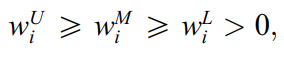•Gurobi Staff

Hi Birkan,

To implement the expression $$\sum_i \sum_{j \neq i} \ln w^L_i - \ln w^U_j$$ as part of the objective function, you would need to define auxiliary variables $$u_i$$ and $$v_j$$ such that $$u_i = \ln w^L_i$$ and $$v_j = \ln w^U_j$$. You can then use the Model.addGenConstrLog() API to implement them:

u = model.addVars(n, name="u")v = model.addVars(n, name="v")for i in range(n):    model.addGenConstrLog(wL[i], u[i], name=f"ln(wL)_{i}")for j in range(n):    model.addGenConstrLog(wU[j], v[j], name=f"ln(wU)_{j}")

The first constraint $$w^L_i + \sum_{j \neq i} w^U_j \geq 1, ~~~ i=1, \cdots, n$$ can be implemented as below:

model.addConstrs(    (wL[i] + gp.quicksum(wU[j] for j in range(n) if j != i) >= 1 for i in range(n)))

You can use the example scripts above to implement your entire model. Please make sure to define finite lower and upper bounds for all variables involved in the general constraints.

Best regards,

Maliheh

•Hi Maliheh,

Firstly, thanks for your answer, it really helps me. I have an other question. I think i wrote rest of the codes correctly. Here its.

import gurobipy as gpfrom gurobipy import GRBmodel = gp.Model()  # Create a Gurobi model instancea=[[(1.0000,1.0000,1.0000),(4.0000,5.0000,6.0000),(2.0000,3.0000,4.0000)],          [(0.1667,0.2000,0.2500),(1.0000,1.0000,1.0000),(0.2500,0.3333,0.5000)],          [(0.2500,0.3333,0.5000),(2.0000,3.0000,4.0000),(1.0000,1.0000,1.0000)]]lij=[[1,4,2],[0.1667,1,0.2500],[0.2500,2,1]]mij=[[1,5,3],[0.2000,1,0.3333],[0.3333,3.0000,1.0000]]uij=[[1,6,4],[0.2500,1,0.5000],[0.5000,4,1]]#VARIABLES FOR WL WM WRW1L=model.addVar(lb=0.0, ub=1.0, vtype=GRB.CONTINUOUS)W1M=model.addVar(lb=0.0, ub=1.0, vtype=GRB.CONTINUOUS)W1U=model.addVar(lb=0.0, ub=1.0, vtype=GRB.CONTINUOUS)W2L=model.addVar(lb=0.0, ub=1.0, vtype=GRB.CONTINUOUS)W2M=model.addVar(lb=0.0, ub=1.0, vtype=GRB.CONTINUOUS)W2U=model.addVar(lb=0.0, ub=1.0, vtype=GRB.CONTINUOUS)W3L=model.addVar(lb=0.0, ub=1.0, vtype=GRB.CONTINUOUS)W3M=model.addVar(lb=0.0, ub=1.0, vtype=GRB.CONTINUOUS)W3U=model.addVar(lb=0.0, ub=1.0, vtype=GRB.CONTINUOUS)#ARRAYS FOR WL WM WUWL=[W1L,W2L,W3L]WM=[W1M,W2M,W3M]WU=[W1U,W2U,W3U]#LN FUNCTIONSWLlogi = model.addVars(3)WUlogi = model.addVars(3)WMlogi = model.addVars(3)WLlogj = model.addVars(3)WUlogj = model.addVars(3)WMlogj = model.addVars(3)lijlog=model.addVars(3)mijlog=model.addVars(3)uijlog=model.addVars(3)for i in range(3):    model.addGenConstrLog(WL[i], WLlogi[i])    model.addGenConstrLog(WM[i], WMlogi[i])    model.addGenConstrLog(WU[i], WUlogi[i])for j in range(3):    model.addGenConstrLog(WU[j], WUlogj[j])    model.addGenConstrLog(WL[j], WLlogj[j])    model.addGenConstrLog(WM[j], WMlogj[j])for i in range (3):    for j in range(3):        model.addGenConstrLog(lij[i][j], lijlog[i][j])        model.addGenConstrLog(mij[i][j], mijlog[i][j])        model.addGenConstrLog(uij[i][j], uijlog[i][j])model.update()#objective functionmodel.setObjective(gp.quicksum((WLlogi[i]-WUlogj[j]-lijlog[i][j])**2+(WMlogi[i]-WMlogj[j]-mijlog[i][j])**2+(WUlogi[i]-WLlogj[j]-uijlog[i][j])**2 for i in range(3) for j in range(3) if i!=j), GRB.MINIMIZE)# Add constraintsmodel.addConstrs((WL[i] + gp.quicksum(WU[j] for j in range(3) if j != i) >= 1 for i in range(3)))model.addConstrs((WL[i] + gp.quicksum(WU[j] for j in range(3) if j != i) <= 1 for i in range(3)))model.addConstrs(gp.quicksum(WM[i] for i in range(3))==1 for j in range(3))model.addConstrs(gp.quicksum(WL[i]+WU[i] for i in range(3))==2 for j in range(3))model.optimize()print(f"Optimal objective value: {model.objVal}")

Now when i try to do for my real number values which is l(i,j),m(i,j) and u(i,j) (obtained from a matrix) gurobi gives the following error.

for i in range (3):    for j in range(3):        model.addGenConstrLog(lij[i][j], lijlog[i][j])        model.addGenConstrLog(mij[i][j], mijlog[i][j])        model.addGenConstrLog(uij[i][j], uijlog[i][j])TypeError: 'Var' object is not subscriptable

Lastly, when i try to make the objective function i get this error. I thinks its because of the u(i,j) m(i,j) and l(i,j)

model.setObjective(gp.quicksum((WLlogi[i]-WUlogj[j]-lijlog[i][j])**2+(WMlogi[i]-WMlogj[j]-mijlog[i][j])**2+(WUlogi[i]-WLlogj[j]-uijlog[i][j])**2 for i in range(3) for j in range(3) if i!=j), GRB.MINIMIZE)TypeError: 'Var' object is not subscriptable

What should i do in this case?

Again thanks for your helps Maliheh.

•Gurobi Staff

Hi Birkan,

The $$l$$, $$m$$, and $$u$$ are known input values to the problem. They are not decision variables.

• You need to remove the variable definitions $$\texttt{lijlog}$$, $$\texttt{mijlog}$$, and $$\texttt{uijlog}$$.
• You also need to remove the general constraint definition related to these parameters.
• When constructing the objective function, you can use the math.log(x) function of Python to represent $$\ln(x)$$ for a real value $$x \geq 0$$.

Furthermore, in your code snippet, you have defined both $$\texttt{WLlogi}$$ and $$\texttt{WLlogj}$$ variables and have added two set of constraints defining the exact same relationship that $$\texttt{WLlog = ln(WL)}$$. The variable $$\texttt{WLlogj}$$ and its associated log constraints are redundant. The same argument applies to variables $$\texttt{WM}$$ and $$\texttt{WU}$$.

Please see the modified script below implementing the original formulation you posted:

import gurobipy as gpfrom gurobipy import GRBfrom math import logmodel = gp.Model()l = [[1, 4, 2], [0.1667, 1, 0.2500], [0.2500, 2, 1]]m = [[1, 5, 3], [0.2000, 1, 0.3333], [0.3333, 3.0000, 1.0000]]u = [[1, 6, 4], [0.2500, 1, 0.5000], [0.5000, 4, 1]]WL = model.addVars(3, ub=1, name="WL")WM = model.addVars(3, ub=1, name="WM")WU = model.addVars(3, ub=1, name="WU")WLlog = model.addVars(3, lb=-GRB.INFINITY)WUlog = model.addVars(3, lb=-GRB.INFINITY)WMlog = model.addVars(3, lb=-GRB.INFINITY)for i in range(3):    model.addGenConstrLog(WL[i], WLlog[i])    model.addGenConstrLog(WM[i], WMlog[i])    model.addGenConstrLog(WU[i], WUlog[i])model.setObjective(    gp.quicksum(        (WLlog[i] - WUlog[j] - log(l[i][j])) ** 2        + (WMlog[i] - WMlog[j] - log(m[i][j])) ** 2        + (WUlog[i] - WLlog[j] - log(u[i][j])) ** 2        for i in range(3)        for j in range(3)        if i != j    ),    GRB.MINIMIZE,)model.addConstrs(    (WL[i] + gp.quicksum(WU[j] for j in range(3) if j != i) >= 1 for i in range(3)))model.addConstrs(    (WU[i] + gp.quicksum(WL[j] for j in range(3) if j != i) <= 1 for i in range(3)))model.addConstr(WM.sum() == 1)model.addConstr(WL.sum() + WU.sum() == 2)model.optimize()print(f"Optimal objective value: {model.ObjVal}")

Best regards,

Maliheh

•Hi Maliheh,

The model worked as it intended to do. Thank you so much for helping.

Best regards.

•Hi Maliheh,

i have problem. The thing that i missed was the last constraint which isHow can i add this one to the constarints. I tried to make them boundries in the variable declaration but i got an error.

Maliheh Aramon